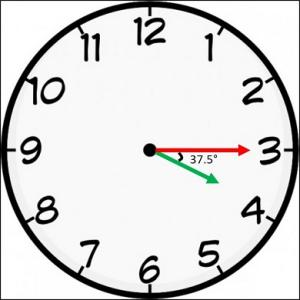# Clocks - Logical Reasoning (MCQ) Questions for Q. 28785

Q.  At what angle are the hands of a clock inclined at 15 minutes past 4?
- Published on 07 Jul 17

a. 32°
b. 37°
c. 32.5°
d. 37.5°

At 4 o’ clock, the hour hand is at 120° from the vertical.

In 15 minutes,

Hour hand = 120 + 15 x (0.5) = 120 + 7.5 = 127.5° (The hour hand moves at 0.5 dpm)

Minute hand = 15 x (6) = 90° (The minute hand moves at 6 dpm)

Difference or Angle between the hands = 127.5 – 90 = 37.5°## ➨ Post your comment / Share knowledgeEnter the code shown above:

(Note: If you cannot read the numbers in the above image, reload the page to generate a new one.)